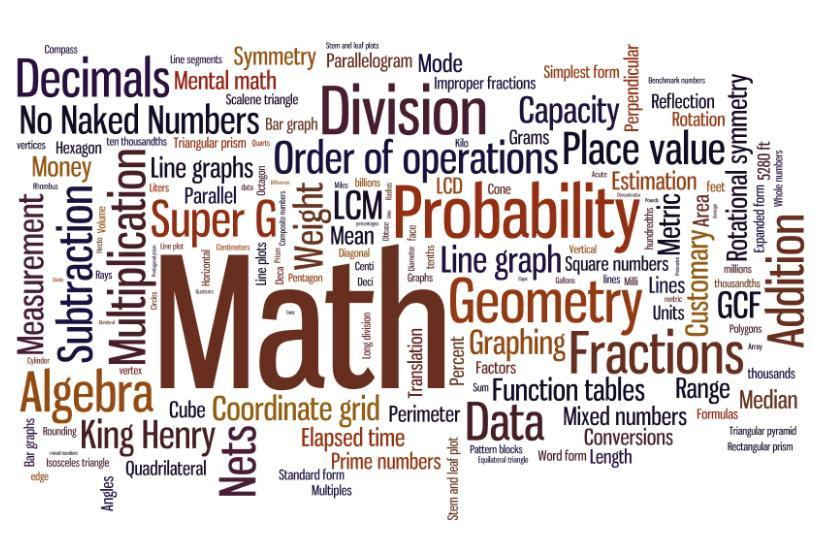# Math (3)

## See if you can answer these math question that range from Algebra to Pre-Calculus

published on October 29, 20166 responses 1 5.0★ / 5

3a+3c
a+2c
8a+2ac
2a+c
ac+a2

c2
xc
(x + c)2
2xc + c2

1
Inffinatly many
8
2
6

### Which of the following expressions is the closest approximation to the height h, in feet, of the roof truss shown below?13
14
15
16
18

15
45
60
30
72

### Ding’s Diner advertised this daily lunch special: “Choose 1 item from each column—only \$4.95!” Thus, each daily lunch special consists of a salad, a soup, a sandwich, and a drink. How many different daily lunch specials are possible?120
8
50
14
30

### In quadrilateral PQRS below, sides PS and QR are parallel for what value of x ?110
70
120
132
150

-9
(9/7)
7
9
(-9/4)

### Which of the following is the sine of angle A in the right triangle below?(5/13)
(12/13)
(13/5)
(12/5)
(5/12)

l = 2w – 3
l = (1/2)w + 3
l = w + 3
l = w – 3
l = 2w + 3

### In the figure below, ∠ ABC ≅ ∠ DFE, ∠ BAC ≅ ∠ FDE, D and F are on AB, AD ≅ FB, and distances in centimeters are as shown. What is the length of AD, in centimeters?1
3
2
4
5

### Each of the variables t, w, x, y, and z represents a different positive real number. Given the equations below, which of the 4 variables w, x, y, and z necessarily has the greatest value?1.23w = t1.01x = t0.99y = t0.23z = t

W
X
Y
Z
Can not be determined